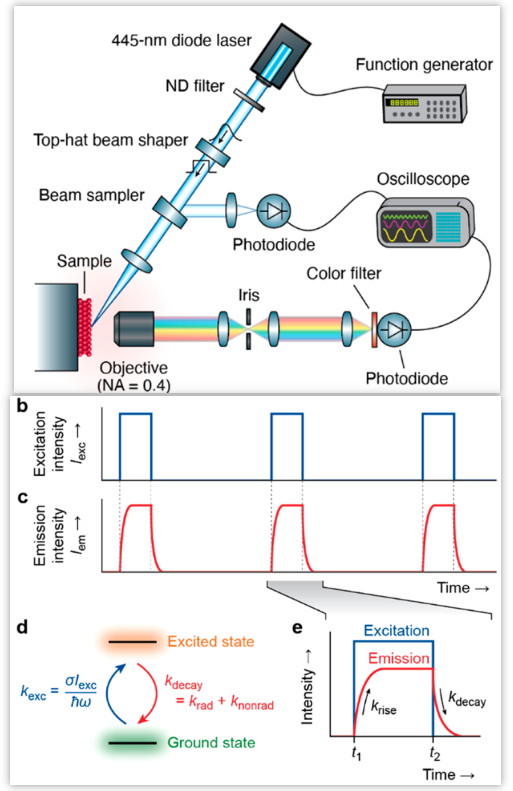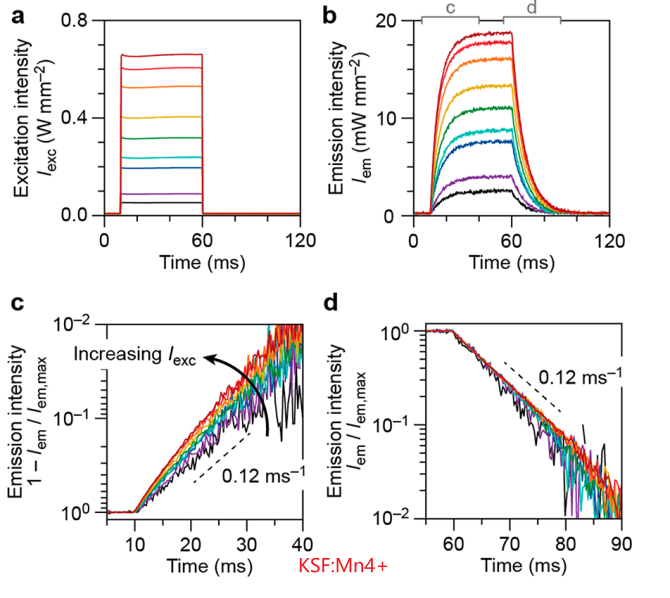## Saturation Mechanisms in Common LED Phosphors

• 商用荧光粉的要求：
• YAG:Ce3+， CaAlSiN3:Eu2+目前还是最好的商用荧光粉，outcompete rapidly developing alternatives。
• 优点：a close to 100% internal quantum yield and prolonged LED operating hours without severe degradation.
• 缺点：thermal quenching or saturation quenching (automotive head lights and projector devices)
• 对于high-power applications应用场景，目前只有YAG:Ce3+满足要求，没有可用的红色荧光粉。Two-level systems $$\frac{\mathrm{d} p}{\mathrm{~d} t}=k_{\mathrm{exc}}[1-p(t)]-k_{\mathrm{decay}} p(t)$$(1) $$p(t)$$ is the probability to find a center in the excited state at some moment in time $$t$$;
(2) $$k_{\mathrm{exc}}=\sigma I_{\mathrm{exc}} / \hbar \omega$$ is the rate constant for excitation, depends on the absorption cross-section $$\sigma$$ of a center, , the excitation intensity $$I_{\mathrm{exc}}$$, , and on the excitation photon energy $$\hbar \omega$$;
(3) $$k_{\text {decay }}$$ is the rate constant for decay, $$=k_{\text {rad }}+k_{\text {nonrad }}$$.

• $$t>t_{2}$$
$$p(t)=p\left(t_{2}\right) e^{-k\tiny{\text {decay }}\left(t-t\tiny{2}\right)}$$
• $$t_{1}<t<t_{2}$$
$$p(t)=p\left(t_{2}\right)\left[1-e^{-k_{\text {rise }}\left(t-t_{1}\right)}\right]$$其中$$k_{\text {rise }}=k_{\text {exc }}+k_{\text {decay }}$$

KSF:Mn4+ Mild experiment conditions
(1) the grains were placed on an indium foil directly connected to a heat sink; (2) the peak excitation intensity was limited to a maximum of 0.7 W mm−2;
(3) the duty cycle was set to no more than 5%;
(4) the heat sink waskept at room temperature.• Mn4+的激发态寿命为8.5 ms，和之前光谱仪测试的一致，而且这里没有随着激发功率的变化而变化，说明没有在这个实验条件下没有open nnradiative decay pathways。
• 激发光为blue，发射光为red，所以必然存在热的生成，但是寿命没有变化，说明在此条件下not sufficient to lead to thermal quenching.
• rise dynamics accelerate with increasing excitation intensity，这很容易理解，因为$$k_{\text {rise }}=k_{\text {exc }}+k_{\text {decay }}$$